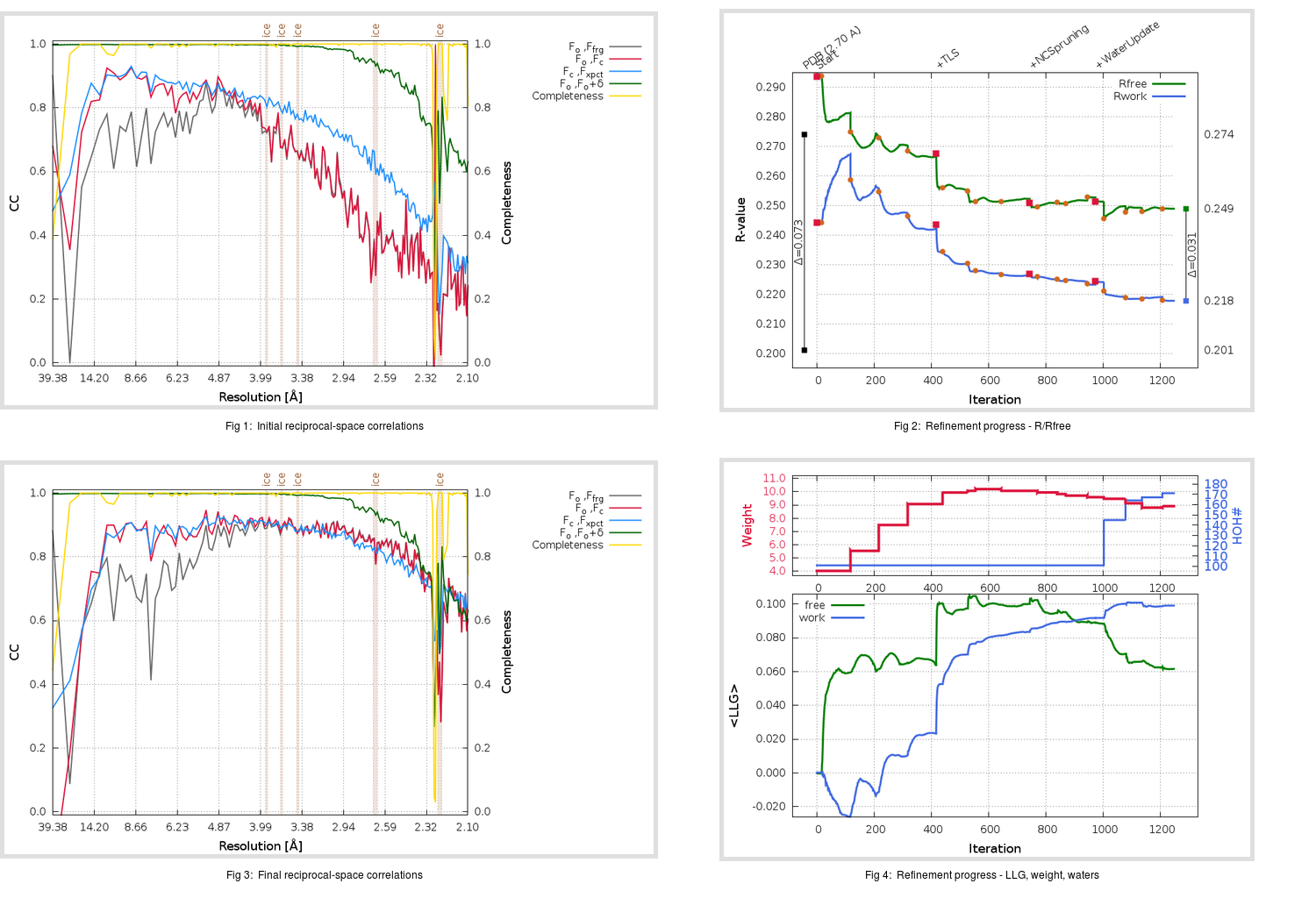Content:

```    Diffraction limits & principal axes of ellipsoid fitted to diffraction cut-off surface:
2.626         0.9154   0.0000   0.4025       0.971 a* + 0.238 c*
2.493         0.0000   1.0000   0.0000       b*
2.521        -0.4025   0.0000   0.9154      -0.290 a* + 0.957 c*
```

## Deposited

` `
 Date deposited Date data collection Resolution R, Rfree 20100310 20060428 2.70 0.2010 0.2740

Molprobity (CCP4 7.0 version) summary:

```Ramachandran outliers =   3.14 %
favored =  84.49 %
Rotamer outliers      =   5.98 %
C-beta deviations     =     0
Clashscore            =  26.62
RMS(bonds)            =   0.0086
RMS(angles)           =   1.52
MolProbity score      =   3.17
Resolution            =   2.70
R-work                =   0.2010
R-free                =   0.2740
```

```Number of waters      =   101

<B> (all atoms) =   49.94 ( sd =   18.13 ) for       4809 non-hydrogen atoms
<B>   (protein) =   50.09 ( sd =   18.24 ) for       4708 non-hydrogen atoms
<B>     (water) =   43.05 ( sd =    9.91 ) for        101 non-hydrogen atoms
<B>    (others) =    0.00 ( sd =    0.00 ) for          0 non-hydrogen atoms

B min/max       (all non-hydrogen atoms) =   13.22 /  126.80
B min/max   (protein non-hydrogen atoms) =   13.22 /  126.80
B min/max     (water non-hydrogen atoms) =   23.46 /   68.66
B min/max     (other non-hydrogen atoms) =    0.00 /    0.00
```

## BUSTER (re-)refinement

` `

Molprobity (CCP4 7.0 version) summary:

```Ramachandran outliers =   1.16 %
favored =  94.22 %
Rotamer outliers      =   9.27 %
C-beta deviations     =     4
Clashscore            =   4.29
RMS(bonds)            =   0.0112
RMS(angles)           =   1.62
MolProbity score      =   2.34
Resolution            =   2.10
R-work                =   0.2179
R-free                =   0.2490
```

```Number of waters      =   171

<B> (all atoms) =   62.94 ( sd =   16.55 ) for       4879 non-hydrogen atoms
<B>   (protein) =   63.11 ( sd =   16.65 ) for       4708 non-hydrogen atoms
<B>     (water) =   58.36 ( sd =   12.67 ) for        171 non-hydrogen atoms
<B>    (others) =    0.00 ( sd =    0.00 ) for          0 non-hydrogen atoms

B min/max       (all non-hydrogen atoms) =   29.37 /  123.52
B min/max   (protein non-hydrogen atoms) =   33.40 /  123.52
B min/max     (water non-hydrogen atoms) =   29.37 /  102.36
B min/max     (other non-hydrogen atoms) =    0.00 /    0.00
```

Refinement progression:Results:

` `
 File Remark 3M3V_aB_refine.01_04_refine.pdb.gz exact refinement commands are in header 3M3V_aB_refine.01_04_refine.mtz.gz including original deposited data and several re-refinement map coefficients 3M3V_aB_refine.01_04_BUSTER_model.cif.gz including any non-standard compound restraints 3M3V_aB_refine.01_04_BUSTER_refln.cif.gz